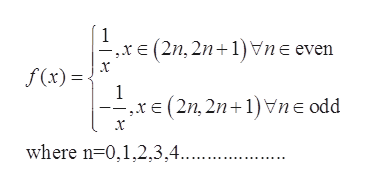Define the function f(x) to be 1/x for x for all x that are (2n,2n+1) for even n and -1/x for x for all x that are you (2n, 2n+1) for odd n. Graph the function and describe for which points limit do not exist. Does the limit exist as x goes to infinity? (The n here represents natural numbers e.g. 0,1,2,...27,... and hence the first interval is (0,1], the second is (1,2], etc.)

Question

Define the function f(x) to be 1/x for x for all x that are (2n,2n+1) for even n and -1/x for x for all x that are you (2n, 2n+1) for odd n. Graph the function and describe for which points limit do not exist. Does the limit exist as x goes to infinity? (The n here represents natural numbers e.g. 0,1,2,...27,... and hence the first interval is (0,1], the second is (1,2], etc.)

Step 1

The given function ishelp_outlineImage Transcriptionclose1 (2n, 21)ne even f(x) 1 (2n, 2n ne odd where n 0,1,2,3,4.... fullscreen
Step 2

To draw the graph for the given function.

To determine the value of limit as x approaches to infinity.

To find the points for which the limit doesn’t exist.

Step 3

The given function ...

Want to see the full answer?

See Solution

Want to see this answer and more?

Our solutions are written by experts, many with advanced degrees, and available 24/7

See Solution
Tagged in

Calculus